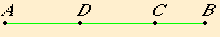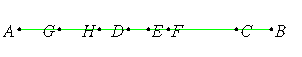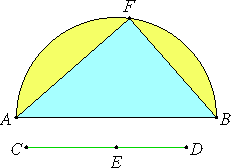Lemma 1.

To find two square numbers such that their sum is also square.

Set out two numbers AB and BC, and let them be either both even or both odd. Then since, whether an even number is subtracted from an even number, or an odd number from an odd number, the remainder is even, therefore the remainder AC is even.

Bisect AC at D. Let AB and BC also be either similar plane numbers, or square numbers, which are themselves also similar plane numbers.Now the product of AB and BC together with the square on CD equals the square on BD. And the product of AB and BC is square, inasmuch as it was proved that, if two similar plane numbers multiplied by one another make some number, the product is square. Therefore two square numbers, the product of AB and BC, and the square on CD, have been found which, when added together, make the square on BD.

And it is clear that two square numbers, the square on BD and the square on CD, have again been found such that their difference, the product of AB and BC, is a square, whenever AB and BC are similar plane numbers. But when they are not similar plane numbers, two square numbers, the square on BD and the square on DC, have been found such that their difference, the product of AB and BC, is not square.

Q.E.D.

Lemma 2.

To find two square numbers such that their sum is not square.

Let the product of AB and BC we said, be square, and CA even. Bisect CA by D.

Lemma 1

It is then clear that the square product of AB and BC together with the square on CD equals the square on BD.

Subtract the unit DE. Therefore the product of AB and BC together with the square on CE is less than the square on BD.I say then that the product of AB and BC together with the square on CE is not square.

If it is square, then it either equals the square on BE, or is less than the square on BE, but cannot be greater lest the unit be divided.

First, if possible, let the product of AB and BC together with the square on CE equal the square on BE, and let GA be double the unit DE.

Since the whole AC is double the whole CD, and in them AG is double DE, therefore the remainder GC is also double the remainder EC. Therefore GC is bisected by E.

II.6

Therefore the product of GB and BC together with the square on CE equals the square on BE

But the product of AB and BC together with the square on CE also, by hypothesis, equals the square on BE, therefore the product of GB and BC together with the square on CE equals the product of AB and BC together with the square on CE.

And, if the common square on CE is subtracted, then it follows that AB equals GB, which is absurd.

Therefore the product of AB and BC together with the square on CE does not equal the square on BE.

I say next that neither is it less than the square on BE.

For, if possible, let it equal the square on BF, and let HA be double DF.

II.6

Now it will again follow that HC is double CF, so that CH is bisected at F, and for this reason the product of HB and BC together with the square on FC equals the square on BF.

But, by hypothesis, the product of AB and BC together with the square on CE also equals the square on BF.

Thus the product of HB and BC together with the square on CF also equals the product of AB and BC together with the square on CE, which is absurd.

Therefore the product of AB and BC together with the square on CE is not less than the square on BE.

And it was proved that neither does it equal the square on BE.

Therefore the product of AB and BC together with the square on CE is not square.

Q.E.D.

Proposition 29

To find two rational straight lines commensurable in square only such that the square on the greater is greater than the square on the less by the square on a straight line commensurable in length with the greater.

Set out any rational straight line AB, and two square numbers CD and DE such that their difference CE is not square.X.6,Cor.

Describe the semicircle AFB on AB, and let it be contrived that DC is to CE as the square on BA is to the square on AF. Join FB.

X.6

Since the square on BA is to the square on AF as DC is to CE, therefore the square on BA has to the square on AF the ratio which the number DC has to the number CE. Therefore the square on BA is commensurable with the square on AF.

X.Def.I.4

But the square on AB is rational, therefore the square on AF is also rational. Therefore AF is also rational.

X.9

And, since DC does not have to CE the ratio which a square number has to a square number, neither does the square on BA have to the square on AF the ratio which a square number has to a square number, therefore AB is incommensurable in length with AF.

Therefore BA and AF are rational straight lines commensurable in square only.

And since DC is to CE as the square on BA is to the square on AF, therefore, in conversion, CD is to DE as the square on AB is to the square on BF.

X.9

But CD has to DE the ratio which a square number has to a square number, therefore the square on AB has to the square on BF the ratio which a square number has to a square number. Therefore AB is commensurable in length with BF.

And the square on AB equals the sum of the squares on AF and FB, therefore the square on AB is greater than the square on AF by the square on BF commensurable with AB.

Therefore there have been found two rational straight lines BA and AF commensurable in square only such that the square on the greater AB is greater than the square on the less AF by the square on BF commensurable in length with AB.

Q.E.D.

Guide

Euclid records in Lemma 1 a method to generate Pythagorean triples. As it depends only on the material in Book IX, logically, it could have appeared there rather than here in Book X. It appears here since it is needed in the proof of the proposition. It is likely much older than the rest of Book X.

Here is a paraphrase of the method of lemma 1, written in a modern algebraic notation. Let n2 (= AB) and m2 (= BC) be two numbers of the same parity with n2 larger. Then n2 – m2 is an even number, so let k (= CD) be its half. Then

n2m2 + k2 = (m2 + k )2.
Therefore,
(nm)2 + ((n2m2)/2)2 = ((n2 + m2)/2)2,

which gives the two square numbers (nm)2 and ((n2 – m2)/2)2 whose sum is also a square.

Pythagorean triples

Three numbers x, y, and z where the sum of squares of the first two is the square of the third form what is now called a Pythagorean triple x : y : z. This lemma shows that if n and m have the same parity and n > m, then n and m generate the Pythagorean triple

 nm : n2 – m22 : n2 + m22

It is easy to see that if x : y : z is a Pythagorean triple, then so is any multiple ax : ay : az of it. A Pythagorean triple that is not a multiple of another Pythagorean triple is called a primitive Pythagorean triple. The numbers n and m are relatively prime if and only if the Pythagorean triple that they generate is primitive. Thus, when n and m are relatively prime odd numbers and n > m, then a primitive Pythagorean triple is generated.

Here’s a table of a few small Pythagorean triples, indexed by n and m. Those that aren’t primitive are colored with a gray background.

13579 1113
3 3 : 4 : 5
5 5 : 12 : 13 15 : 8 : 17
7 7 : 24 : 25 21 : 20 : 29 35 : 12 : 37
9 9 : 40 : 41 27 : 36 : 45 45 : 28 : 53 63 : 16 : 65
11 11 : 60 : 61 33 : 56 : 65 55 : 48 : 73 77 : 36 : 85 99 : 20 : 101
13 13 : 84 : 85 39 : 80 : 89 65 : 72 : 97 91 : 60 : 109 117 : 44 : 125 143 : 24 : 145
15 15 : 112 : 113 45 : 108 : 117 75 : 100 : 125 105 : 88 : 137 135 : 72 : 153 165 : 52 : 173 195 : 28 : 197